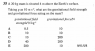# Gravitational field strength of a mass

• Tulatalu

#### Tulatalu

User informed about mandatory use of the homework template.
A 20 kg mass is situated 4m above the Earth's surface
Taking g as 10 m/s2. What are the gravitational field strength and gravitational force acting on the mass?

Am I wrong when I say that because the height of the mass ( which is 4m) is too little comparing with the radius of the Earth so that the gravitational field strength is equal to the free fall acceleration? My answer key said that not 10 but 40 is the right answer. Can anybody explain to me why the answer is 40?

Hi Tulatalu,

Please make use of the homework template when you post in the homework forums. Its purpose is to make it easier for us to help you.

First of all, you are missing units in your answer. Units are important in physics and your answer does not have any meaning without them. Therefore, you cannot just say 10, you need to specify 10 what. How would you answer the following questions? What is the definition of the gravitational field strength? What is the relation between the gravitational field strength and the gravitational force? What units are gravitational field strength and gravitational force measured in?

if g is 10 m/s/s, then its not the earth

if g is 10 m/s/s, then its not the earth
It can very well be Earth. It depends on the precision with which the number is given. Without decimals, 10 m/s2 is the best approximation of Earth gravity and this value is often used to give an easy first estimate. Also, I do not see how this comment helps the OP deal with the problem.

how can you expect to be accurate then ?

You are accurate to roughly the accuracy with which the input is given. Besides, this problem is mainly conceptual and explicitly states that you should use 10 m/s2.

I'm sorry. There are symbols and formula that I don't how to text them.
Gravitational field strength = (GM/r/r)
Hope someone can explain them to me why the g.f.s is equal to 40 m/s/s in the problem above

There are symbols and formula that I don't how to text them.
Either there's something wrong with the answer or you have not conveyed the question correctly.
Can you post an image of the question?

Either there's something wrong with the answer or you have not conveyed the question correctly.
Can you post an image of the question?
There you are.

#### Attachments

•Capture.PNG
6.1 KB · Views: 402

Then it is clear that the answer key is simply wrong. It should say C, not D.

Thanks. I'm just not confident enough to say so :D

It is D
As haruspex said, it is C. It cannot be D since g is the gravitational field strength and therefore must be 10 m/s^2.

•scott mcleod jr and Tulatalu
It is important to think of what the difference between field strength and force is.
If you first think of what the mathematical formula of a force is you can figure out the second one.
The first one can be more confusing but if you pay close attention to the units (try writing them out) you can determine what it is they are looking for.

I teach general through AP Physics and termonology is probibly the thing that causes students the most dificulty, sometimes solving a problem involves some detective work, so if you don't at first understand the termonology, next look at things like units and equations there are many paths to finding solutions in physics, the important thing to remeber is there are some absolute truths (what a force is, what a field is and how they interact and what units you have to have for certain things) if you can remember these things the things you can't remember or don't understand you can find out through logical arguments. Very few physicists remember every formula or idea instead they remember basic truths and using these you can usually work your way back to what you don't know.

•Tulatalu
The gravitational field strength of the Earth is g.
The Universal Gravitational Constant is G.
g in your problem is acceleration (m/s^2)

If you calculate the gravitational field strength near the surface of the Earth utilizing G you get g, or approximately 10 m/s/s. The Earth is so large and its mass is so great that being just a small distance from the surface or being an object of relatively small mass (compared to the earth) has practically no effect on the gravitational field strength. but forces rely on masses being accelerated, soo... if Force = mass * acceleration and our acceleration is g (or 10 m/s/s) then your force is the mass (20kg) * g (10 m/s/s) or 200 N

•Tulatalu
Physics teachers make mistakes all the time, the good ones like it when you point out that their answer is incorrect. :)

Physics teachers make mistakes all the time, the good ones like it when you point out that their answer is incorrect. :)
I'm studying A level Physics myself and they are quite different from Physics in my own langluage so I am not really confident. :D

•scott mcleod jr